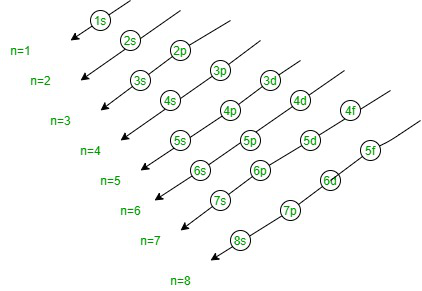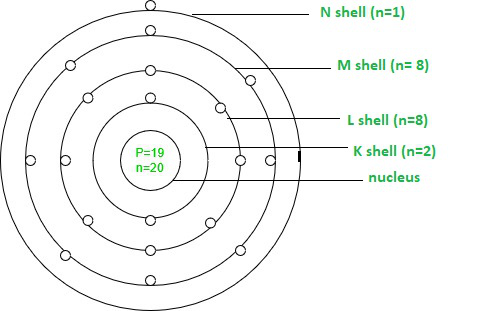Open in App
Not now

# Electronic Configuration of Elements

• Last Updated : 03 Sep, 2021

We already know that electrons orbit the nucleus in an atom, while protons and neutrons are contained within it. How do electrons revolve, is the question? Do they appear to be moving at random? Is there a set path they take? What’s the best way to put them together? Electronic Configuration is the answer to all of these queries. Let’s have a look at how electrons are arranged around a nucleus.

### Distribution of Electrons in Different Orbits

The planetary model of an atom was created by Neils Bohr. He was the first to indicate that the properties of the elements have a regular pattern. The electronic structure of an atom is based on the “Bohr atomic model.” He was the first to describe the arrangement of electrons in distinct orbits/shells (electronic configuration). He proposed that electrons are spread in electronic shells that are circular in shape (orbits). These electrons travel a defined distance around the nucleus in orbits.

Before understanding the concept of Electronic Configuration, lets first understand some basic terms used with it as mentioned below:

• Shells: It is based on the principal quantum number (n)  which determines the maximum number of electrons that can be placed in a shell. The number of electrons in each shell is given by the formula 2n2 where n represents shell number.
• Subshells: It is based on an azimuthal quantum number which is widely denoted by ‘I’.  At the same time, the azimuthal quantum number depends on the value of the principal quantum number (n). For example, if the value of shell n=3. The subshells corresponding to it will be s,p, and d with I=0, I=1, and I=2. These are the shells in which the actual distribution of electrons takes place. And the maximum number of electrons accommodated by a sub-shell is given by the formula 2(2I+1). The possible subshells and their resulting electronic configuration based on quantum number are given below.

### Electronic Configuration

The representation of electrons distributed in atomic shells of an element is known as electronic configuration. The electrons are mathematically located in these subshells and the notations help in locating the position of these electrons as well as the electronic configuration.

An effective study of these electronic configurations written with specific notation can provide details about the particular element. For example, the specific notation for germanium (Ge) would be,1s2 2s2 2p6 3s2 3p6 4s2 3d10 4p2 .

Notation of Electronic Configuration:

Notation is the representation of the number of electrons present in the subshell. It is written with the shell number, name of the subshell, and a total number of electrons present in the subshell in superscript.

For example, the electronic configuration of oxygen can be written as 1s2 2s2 2p4.

The below table consists of the electronic configuration of the first 20 elements.

### Principles for Filling the Shells

Following are the rules or principles that help to fill the shells of an atom:

• Aufbau Principle: The principle states that the electrons will occupy the orbits with lower energy and then the orbits of higher energy levels. The principle gets its name from the Greek word “Aufbeen” which means building up. As per the following principle the electrons are arranged in the order as,• Pauli Exclusion Principle: The Pauli Exclusion Principle states that the maximum of two electrons can fit into an orbit with opposite spins. It is based on the concept that if two electrons carry equal azimuthal numbers then they will have opposite spins in the orbit.
• Hund’s Rule: The rule states that every orbit is initially occupied by a single electron before a second electron fills the orbit. It’s the rule that determines the order of electrons in the sub-shell.

### How to write the electronic configuration of elements?

So, before drawing an electronic configuration we need to extract some information from the periodic table like the atomic number, no. of electrons, shells, etc. Looking through an example let’s understand the writing method of an electronic configuration. The potassium element has atomic number 19. And has 19 electrons which will be placed in s and p sub-shell.

The electronic configuration can be written as 1s2 2s2 2p6 3s2 3p6 4s1. Its 19 electrons can be divided into different shells in a manner as:

• K shell (n=1)= 2,
• L shell (n=2) =8,
• M shell (n=3)=8, and
• N shell (n=4)=1.Electronic Configuration of Potassium

### Sample Questions

Question 1: Write down the electronic configuration of Aluminum.

The electronic configuration of Aluminum (Al) is 1s2 2s2 2p6 3s2 3p1 in spdf notation and 2, 8, 3 in orbital diagram.

Question 2: List the significance of electronic configuration.

The significance are mentioned below:

1. They help to know the reactivity state of the atom.
2. It helps to determine its physical as well as chemical properties.
3. It predicts an atom’s magnetic behavior, etc.

Question 3: Write down the electronic configuration of ideal gases.

Following are the electronic configuration of some ideal gases:

• Helium (He) ⇒ 1s2
• Neon (Ne) ⇒ 1s2 2s2 2p6
• Argon (Ar) ⇒ 1s2 2s2 2p6 3s2 3p6
• Krypton (Kr) ⇒ 1s2 2s2 2p6 3s2 3p6 3d10 4s2 4p6
• Xenon (Xe) ⇒ 1s2 2s2 2p6 3s2 3p6 3d10 4s2 4p6 4d10 5s2 5p6
• Radon (Rn) ⇒  1s2 2s2 2p6 3s2 3p6 3d10 4s2 4p6 4d10 5s2 5p6 4f14 5d10 6s2 6p6

Question 4: How does copper disobey the Aufbau principle?

Since, the electronic configuration of Copper is 1s2 2s2 2p6 3s2 3p6 3d10 4s1. The violation of the Aufbau principle is due to the energy gap between 3d and 4s orbitals. As the completely filled d-orbital offers more stability than the partially filled configuration.

Question 5: How many electrons does each of the sub-shells hold?